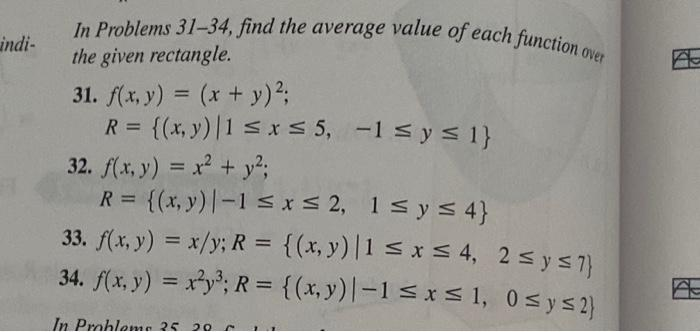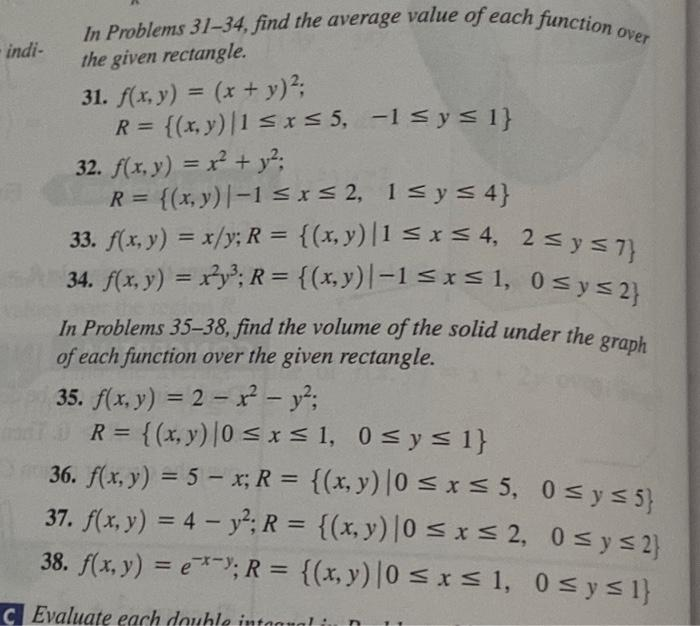Home / Expert Answers / Calculus / in-problems-31-34-find-the-average-value-of-each-function-over-the-given-rectangle-31-f-x-y-pa973

# (Solved): In Problems 31-34, find the average value of each function over the given rectangle. 31. f(x, y) = ( ...

In Problems 31-34, find the average value of each function over the given rectangle. 31. f(x, y) = (x + y)²; R = {(x, y) |1 ≤ x ≤ 5, -1 ≤ y ≤ 1} 32. f(x, y) = x² + y²; = {(x, y) | -1 ≤ x ≤ 2, 1 ≤ y ≤ 4} 33. f(x, y) = x/y; R = 34. f(x, y) = x²y³; R = In Problems 35 20 {(x, y) |1 ≤ x ≤ 4, 2 ≤ y ≤7} {(x,y) | -1 ≤ x ≤ 1, 0≤ y ≤ 2} please help with 31,33,35, and 37In Problems 31-34, find the average value of each function over the given rectangle. 31. 32. 33. 34. In Problems 31-34, find the average value of each function over the given rectangle. 31. 32. 33. 34. In Problems 35-38, find the volume of the solid under the graph of each function over the given rectangle. 35. ; 36. 37. 38.

We have an Answer from Expert